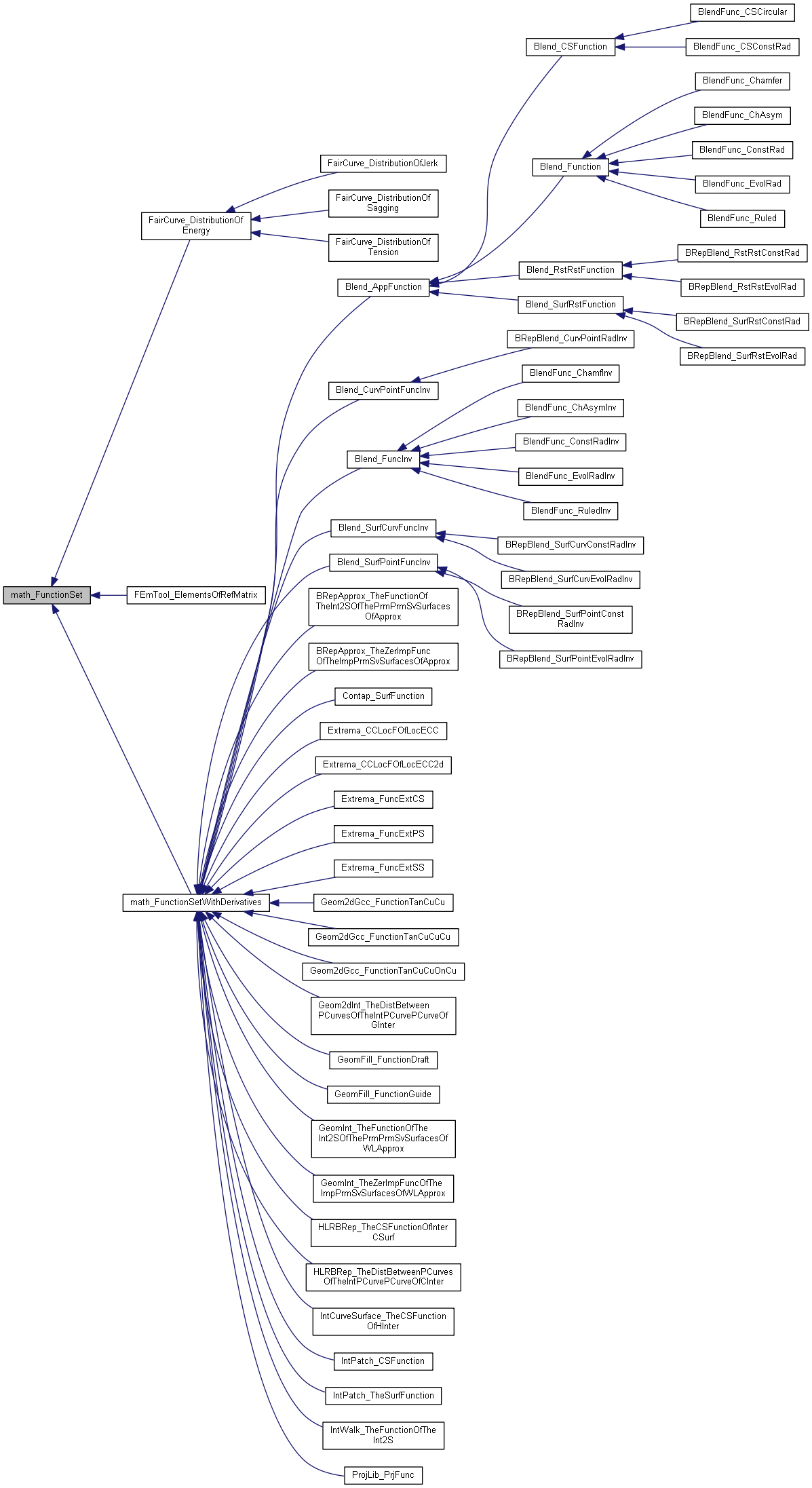math_FunctionSet Class Referenceabstract

This abstract class describes the virtual functions associated to a set on N Functions of M independant variables. More...

`#include <math_FunctionSet.hxx>`

Inheritance diagram for math_FunctionSet:[legend]

## Public Member Functions

virtual Standard_Integer NbVariables () const =0
Returns the number of variables of the function. More...

virtual Standard_Integer NbEquations () const =0
Returns the number of equations of the function. More...

virtual Standard_Boolean Value (const math_Vector &X, math_Vector &F)=0
Computes the values <F> of the functions for the variable <X>. returns True if the computation was done successfully, False otherwise. More...

virtual Standard_Integer GetStateNumber ()
Returns the state of the function corresponding to the latestcall of any methods associated with the function. This function is called by each of the algorithms described later which define the function Integer Algorithm::StateNumber(). The algorithm has the responsibility to call this function when it has found a solution (i.e. a root or a minimum) and has to maintain the association between the solution found and this StateNumber. Byu default, this method returns 0 (which means for the algorithm: no state has been saved). It is the responsibility of the programmer to decide if he needs to save the current state of the function and to return an Integer that allows retrieval of the state. More...

virtual ~math_FunctionSet ()

## Detailed Description

This abstract class describes the virtual functions associated to a set on N Functions of M independant variables.

## Constructor & Destructor Documentation

 virtual math_FunctionSet::~math_FunctionSet ( )
virtual

## Member Function Documentation

 virtual Standard_Integer math_FunctionSet::GetStateNumber ( )
virtual

Returns the state of the function corresponding to the latestcall of any methods associated with the function. This function is called by each of the algorithms described later which define the function Integer Algorithm::StateNumber(). The algorithm has the responsibility to call this function when it has found a solution (i.e. a root or a minimum) and has to maintain the association between the solution found and this StateNumber. Byu default, this method returns 0 (which means for the algorithm: no state has been saved). It is the responsibility of the programmer to decide if he needs to save the current state of the function and to return an Integer that allows retrieval of the state.

Reimplemented in Extrema_FuncExtPS, Extrema_FuncExtCS, Extrema_CCLocFOfLocECC, Extrema_CCLocFOfLocECC2d, and Extrema_FuncExtSS.

 virtual Standard_Boolean math_FunctionSet::Value ( const math_Vector & X, math_Vector & F )
pure virtual

The documentation for this class was generated from the following file: